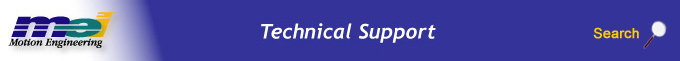.

# PID Parameter Definitions

 Kp Proportional Gain. Increasing proportional gain will decrease position error. Excessive amount of proportional gain will cause middle frequency oscillations (~10 - 100 Hz). (DAC counts / error counts) Ki Integral Gain. Closes steady state position errors. Excessive amount of integral gain will cause large, slow (~ <10 Hz) oscillations. (DAC counts / (error counts * sample period)) Kd Derivative Gain. Damps out low frequency oscillations. Excessive derivative gain will cause high frequency squealing, screeching, or high frequency (~ > 100 Hz) oscillations. (DAC counts / (error counts / sample period)) Kpff Position Feed Forward Gain. Compensates for position-based effects in a system. Its effect is similar to having a spring attached to the stage. (DAC counts / position counts) Kvff Velocity Feed Forward Gain. Compensates for velocity-based friction or similar effects in a system. (DAC counts * (commanded position counts / sample period)) Kaff Acceleration Feed Forward Gain. Compensates for mass in a system. (DAC counts / (commanded position counts / sample period2 )) Kfff Friction Feed Forward Gain. Compensates for Friction in a stage. (DAC counts) IMaxMoving Integral Limit During Motion. The integral is clipped to this value while the motion is taking place. The motion is determined in motion that's based on commanded velocity. If this is set to zero, the integral is effectively turned off during motion. A common way to enhance stability is to set ImaxMoving to half of the Output Limit (if it is used). Setting ImaxMoving to zero can reduce settling time in non-ideal systems. (DAC counts) IMaxRest Integral Limit During Rest. The integral is clipped to this value while the motion is idle. The motion is determined in motion that's based on commanded velocity. If this is set to zero, the integral is effectively turned off when there is no motion. A common way to enhance stability is to set ImaxRest to half of the Output Limit (if it is used). (DAC counts) DRate Derivative Sub Sampling. The parameter causes the derivative term to be evaluated every DRate + 1 samples. This parameter is normally zero. If an encoder has very low resolution, DRate can have a smoothing effect, as if a higher resolution encoder is being used. (sample periods - 1) Output Limit Control Loop Output Limit. The absolute value of the control output will never exceed this value. This is commonly used to limit current that's going to a motor. The more negative value contained between Output Limit and Output Limit High will take precedence. The more positive value contained between Output Limit and Output Limit Low will take precedence. (DAC counts) Output Limit High Control Loop Output Positive Limit. The control output will never exceed this value in the positive sense. The smaller (more negative) value contained between Output Limit and Output Limit High will take precedence. (DAC counts) Output Limit Low Control Loop Output Negative Limit. The control output will never exceed this value in the negative sense. The smaller (more positive) value contained between Output Limit and Output Limit Low will take precedence. (DAC counts) Output Offset Control Loop Offset. Creates an offset to the control output. This is useful for counter acting gravity on vertical stages. The Output Offset is not removed from the DAC in an Abort condition. (DAC counts) Ka0 Used only for noise injection during testing. Ka1 Currently not used. Ka2 Currently not used.

 Legal Notice  |  Tech Email  |  Feedback        Copyright © 2001-2010 Motion Engineering